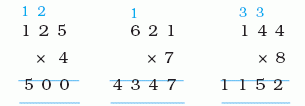Name: ___________________Date:___________________

 Email us to get an instant 20% discount on highly effective K-12 Math & English kwizNET Programs!

Grade 4 - Mathematics3.28 Multiplying One Digit and Three Digit Number

 Multiply the single digit number by each digit of the three digit number. Multlply by ones, tens and hundreds. Multiplication without regroupingMultiplication with regroupingDirections: Find the products of the following. Also write at least 10 examples of your own.Name: ___________________Date:___________________

Grade 4 - Mathematics3.28 Multiplying One Digit and Three Digit Number

 Question 1: 9 * 680

 Question 2: 7 * 173

 Question 3: 9 * 183

 Question 4: 5 * 294

 Question 5: 2 * 973

Question 6: This question is available to subscribers only!

Question 7: This question is available to subscribers only!

Question 8: This question is available to subscribers only!

Question 9: This question is available to subscribers only!

Question 10: This question is available to subscribers only!